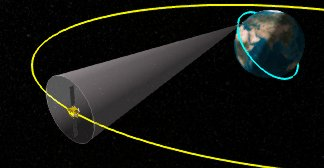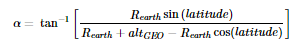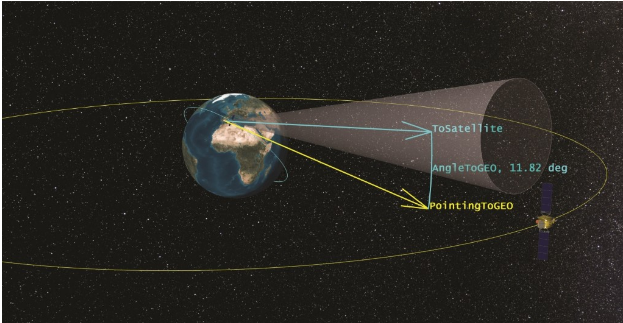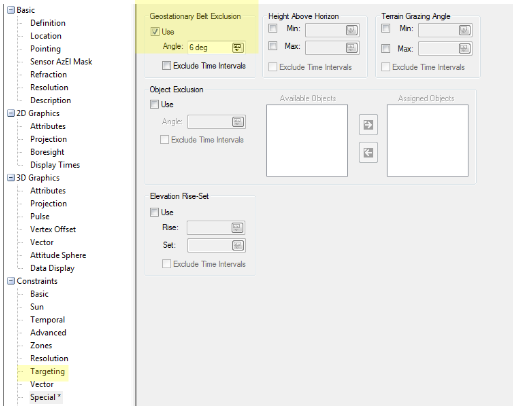Back

# Could my Communications to LEO be Interfering at GEO?

There are a lot of satellites out at Geosynchronous orbit with large dishes collecting anything that happens to be radiating from Earth in their direction. For LEO satellite operators communicating with their assets like this can lead to inadvertent interference on these GEO satellites or even intercepted communications by the GEO satellites.<How do you prevent this from happening? How do you determine when your pointing angle to your LEO asset also intersects the GEO belt? Using STK Analysis Workbench, you can follow these easy steps:

2. Create an arbitrary GEO satellite.
3. Create a plan that is normal to the GEO satellite's angular momentum vector.
4. Create a vector that is the projection of the vector created in step one (1) onto the plane that was created in step three (3).
5. Create an Aligned and Constrained coordinate system using the vector created in step 4 as the aligned vector and the Earth ICRF z-vector as the constrainted vector.
6. Create a fixed in axes vector within the coordinate system from step 5 with right ascension zero (0) and a declination of α (α = the angle pointing to the GEO belt from your facility as a function of latitude.REarth = Radius of Earth and altGEO = Geosynchronous altitude

1. Create an angle between the vector created in step 1 above and the vector created in step 6 above.
2. Use the angle constraint (under the Vector constraints tab) to set this angle to be a minimum value (no access when this angle gets below this minimum value).<

What if you don’t want to do all this math and set up the vectors? Well, STK makes it easy for you with the built in GEO exclusion constraint (Special constraints tab, Geostationary Belt Exclusion).For more on the Geostationary Belt Exclusion constraint see the AGI help.

#### Analysis Workbench

Create custom functions and calculations relative to times, positions, and reference frames.